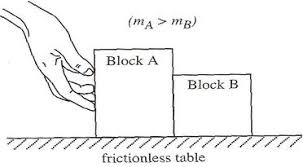# Normal reaction doubt

• Vatsal Goyal

## Homework Statement

My question is not a homework problem. I am having difficulty in understanding the concept oof contact forces.

## Homework Equations

What I don't understand is that when two bodies A and B are in contact and left on a frictionless surface, they apply normal reactions on each other, then why doesn't that normal reaction force give some acceleration to the blocks and they move away from each other?

## The Attempt at a Solution

The only viable solution I can come up with is that the normal reaction is zero, but they why it would be zero I don't know.

## Homework Statement

My question is not a homework problem. I am having difficulty in understanding the concept oof contact forces.

## Homework Equations

What I don't understand is that when two bodies A and B are in contact and left on a frictionless surface, they apply normal reactions on each other, then why doesn't that normal reaction force give some acceleration to the blocks and they move away from each other?

## The Attempt at a Solution

The only viable solution I can come up with is that the normal reaction is zero, but they why it would be zero I don't know.
When you say they "are in contact and left on a frictionless surface", do you mean that they are moving at constant velocity or accelerating or what? Consider the picture shown below. Let's say that the hand exerts constant force F to the right. The two masses will accelerate to the right with acceleration ##a=F/(m_A+m_B)##.
Block A experiences two horizontal forces, one from the hand and one from Block B. Block B experiences only one horizontal force and that is the contact force exerted on it by block A. It is that contact force that accelerates block B. Now suppose that the hand stops pushing when the blocks reach speed v. Do you see why the contact force is zero after the hand stops pushing?#### Attachments

the normal reaction is zero, but they why it would be zero
It helps to start with a definition of normal force: it is that force of minimum magnitude necessary to inhibit interpenetration of the objects. In the absence of any force tending to push one into the other there is therefore no normal force.

Last edited:
•Vatsal Goyal